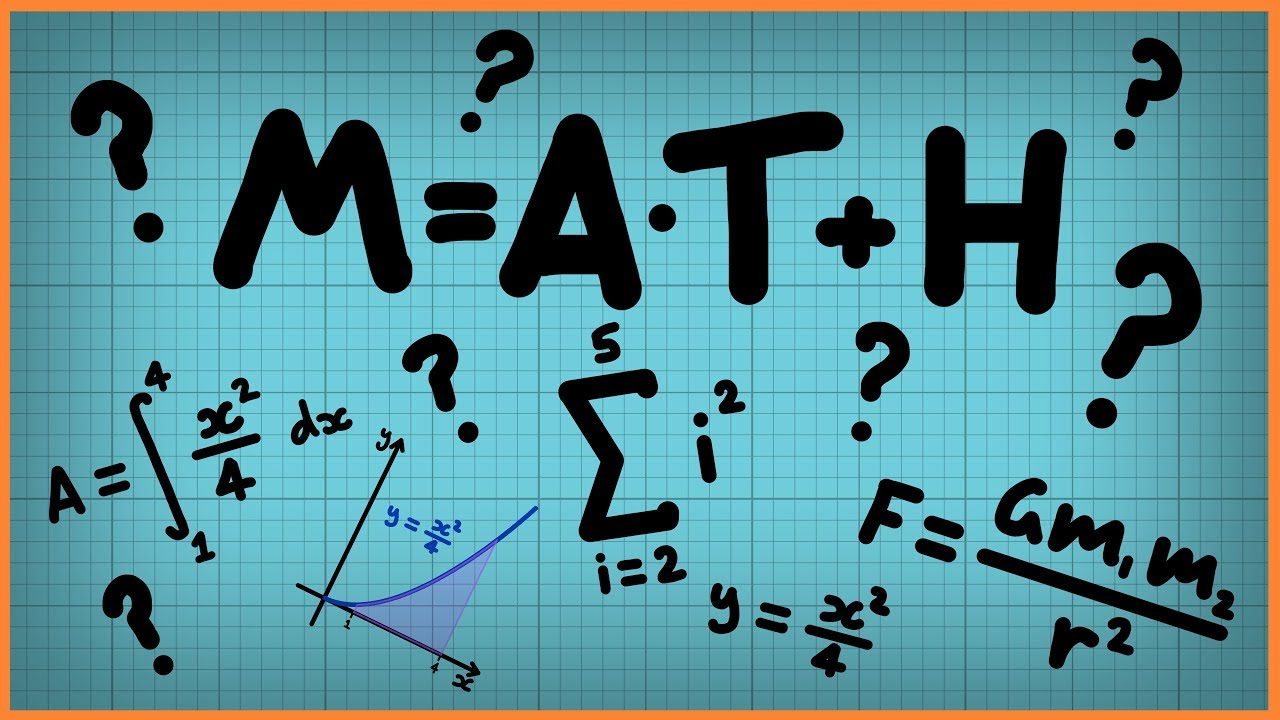一般的運算

加法、減法、乘法、除法a = 3 + 2;
b = 3 - 2;
c = 3 * 2;
d = 3 / 2;
print(a);
print(b);
print(c);
print(d);

e = 15 % 2;
f = 12 % 3;
g = 93 % 6;
print(e);
print(f);
print(g);

i = 3 ** 4;
print(i);

改變一個變數

a = 3;
print(a);

b = a * 3;
a = b;
print(a);

a = 3;
print(a);
a = a * 3;
print(a);

a = 3;
print(a);
a *= 3;
print(a);

a += 3;
a -= 3;
a *= 3;
a /= 3;
a %= 3;

想想看

a, b, c, d, e = 54;
a += 3;
b -= 3;
c *= 3;
d /= 3;
e %= 7;

+1